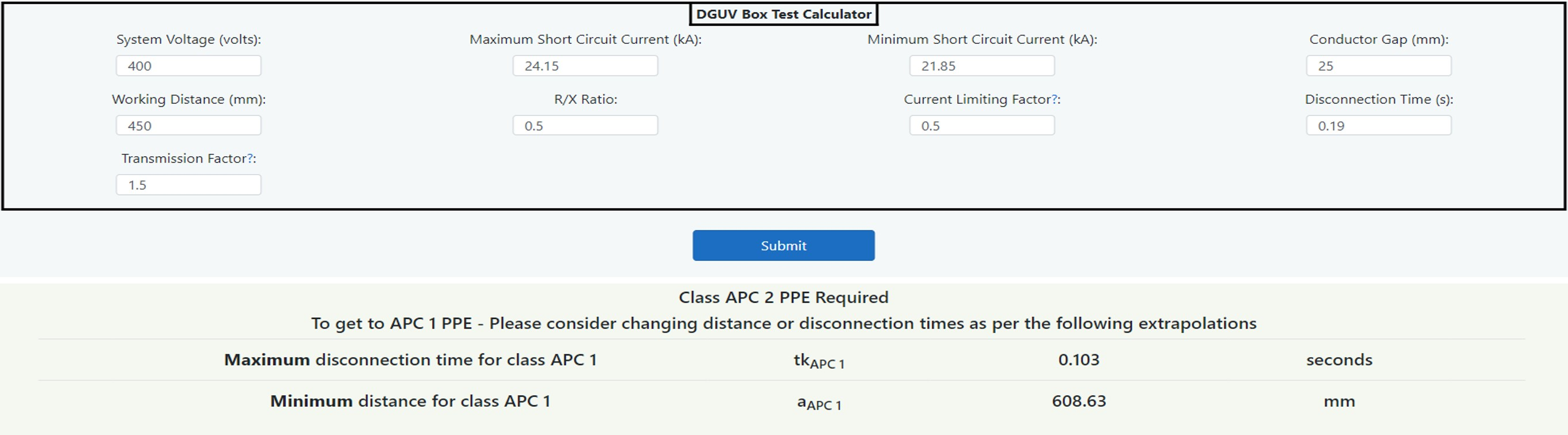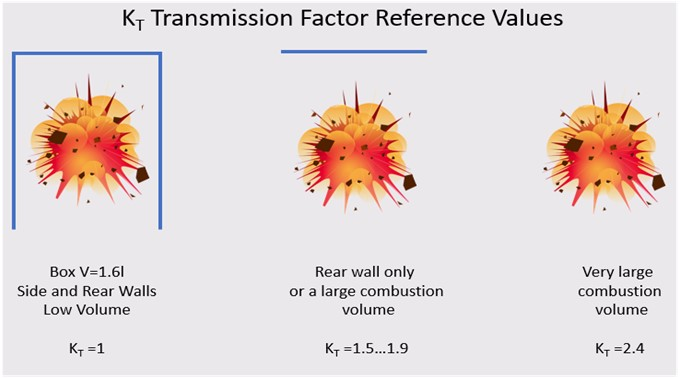# DGUV Box Test Calculator Guide (Level 1)

### What is it?

This calculator in based upon the DGUV-I-203-5188 E Guide to the Selection of Personal Protective Equipment for Electrical Work. It is derived from the standard IEC 61482-1-2: Live Working - Protective Clothing Against the Thermal Hazards of an Electric Arc Method 2: Determination of arc protection class of material and clothing by using a constrained and directed arc (box test). Further information is available in Chapter 14: Hazard and Severity Calculators.

### Why would you use it?

This calculator can be used where there is a requirement to provide PPE that has been tested to the standard IEC 61482-1-2 described above.

### Where would you use it?

The calculator is based upon published white papers and so does not carry the same weight as IEEE 1584:2018. As such it can be used as a guide with good engineering judgement applied in respect of input values and results. The calculator has limitations; it cannot be applied to every situation, and it is recommended that the following limitations are applied:

• Voltage range 50 volts to 1,000 volts. (Upper limit advised)
• A minimum short circuit current value less than the maximum short circuit current.
• AC (Alternating Current) Faults only.
• Instead of creating an incident energy level in cal/cm2 the calculator will produce an arc power based upon the physics of the arcing current.

Whilst the DGUV guide is used in parts of Europe for low voltage utility applications, it is recommended that IEEE 1584 is used for industrial and commercial purposes. There is more information about this standard and the DGUV Box Test Algorithm in Chapter 7: Protection which gives a detailed comparison to IEEE 1584 and Chapter 16: Rules, Codes and Legislation.

### How do you use it?

The following sets out how a competent electrical engineer can use the calculator to arrive at an arc protection class of PPE tested and certified to the box test requirements at a stated working distance. This should be read and applied in accordance with the German publication DGUV-I-203-5188 E Guide to the Selection of Personal Protective Equipment for Electrical Work.##### System Voltage (volts) - Data Entry
The three-phase line-to-line voltage in volts.

##### Maximum & Minimum Short Circuit Current (I”k3max I”k3min) in kA - Data Entry
A maximum and a minimum short circuit current is required in accordance with German standard VDE 0102:2016 short circuit currents in three-phase AC systems. This is required in order to calculate a minimum short circuit current in order to determine the minimum arcing current. The standard is based upon DIN EN 60909 short circuit currents in three-phase AC systems. The standard DGUV-I-203-5188 E states that “calculation software is usually available for this process”. This is to obtain maximum and minimum prospective three-phase initial short circuit AC currents I”k3max and I”k3min. However, for single circuits at low voltage, the minimum and maximum short circuit currents are given in this guide in the prospective short circuit current calculator.

##### R/X Ratio - Data Entry
An R/X ratio is required which is not always easy to establish. However, the R/X can be found from the prospective short circuit current calculator as a reciprocal of the X/R value for low voltage circuits fed by a transformer.

##### Electrode Gap in mm (d) - Data Entry
This is the minimum gap between the conductors measured in millimetres.

##### Current Limiting Factor (KB) - Data Entry
The current limiting factor is simply applied to the minimum short circuit current I”k3min to produce an arcing current.

##### Minimum Arcing Current in kA (IkLB) - Calculated
This is calculated from the current limiting factor and minimum short circuit current.

##### Disconnection Time in seconds (tk) - Data Entry
This is the time to clear the minimum arcing current above and is obtained from the time current curve of the upstream protective device. See Chapter 4: Prediction.

##### Short Circuit Power in MVA (S”k) - Calculated
This is simply the maximum available power based upon the maximum short circuit current I”k3max and line voltage. (√3 VI"k3max)

##### Normalised Arc Power Kp - Calculated
The normalised electric power is a factor which accounts for the arc formation and geometry of the conductors. For worst case conditions it is calculated from the following equation:

kp_max =
 0.29 (R/X)0.17

##### Electric Arc Power PLB (MW) - Calculated
Electric arc power Is the power transferred to the arc based upon the Short Circuit Power (S”k) times the normalised electric power (Kp).

##### Arc Energy (Anticipated Value) WLB - Calculated
The anticipated value of arc energy takes into account the electrical arc power and the disconnection time. (WLB = PLB x tk)

##### Arc Protection Class of PPE WLBP_APC1 & WLBP_APC2 - Given Values
The arc protection class of PPE is given from the DGUV guide, and the latest figures are WLBP_APC1 = 168 kJ and WLBP_APC2 = 320 kJ. This value is the protection level of APC1 or 2 at a distance of 300mm. (The sample set up distance for the box testing of PPE)

##### Transmission Factor KT - Data Entry
The transmission factor is derived from whether the arc is enclosed (in a box), semi enclosed, in air but against a rear wall or in open air. A diagram of the three configurations is shown in Figure 14.22.##### Working Distance (a) - Data Entry
Distance in millimetres from the arc to the person’s body (torso).

##### Protection Level of Clothing WLBS_APC1 and WLBS_APC2 - Calculated
Protection level of the clothing is obtained by extrapolation of the box test parameters to the arc location. It is as follows:

WLBS = KT x (
 a 300
) 2 x WLBP

##### PPE Selection
This is the final step which is to compares arc energy (anticipated value) WLB with the protection level of the clothing WLBS. Protection is given if the following is true:

WLBS ≥ WLB